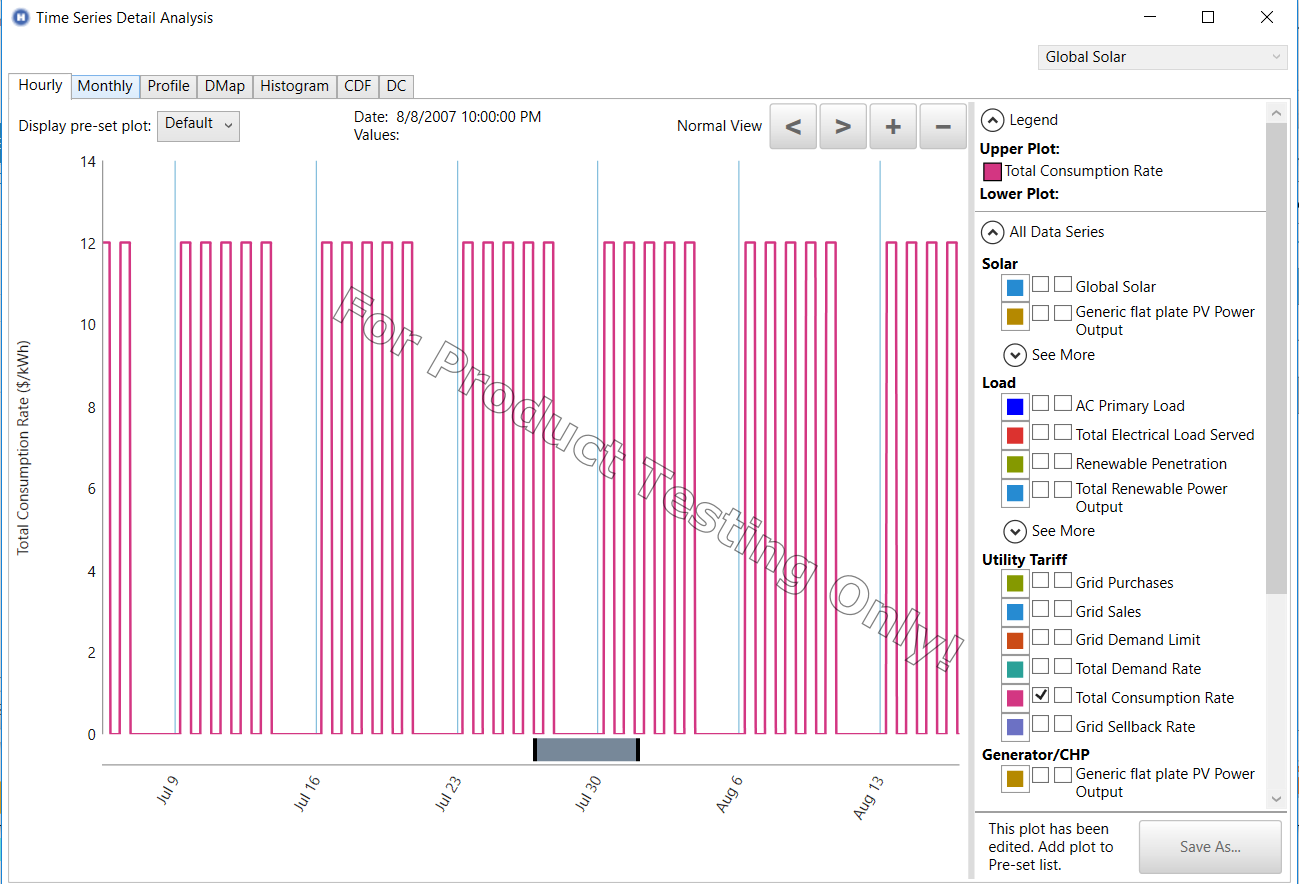# HOMER Grid 1.4

 Time Series Data Series

##This article provides an explanation of a few of the data series found in the Time Series plot.

Total Consumption Rate

Grid Sellback Rate

## Total Consumption Rate

The total consumption rate is a data series which consolidated all the consumption prices for the year based on grid purchases. So, this would include consumption rates of the type “NET, Net Purchase and Import”. The total consumption rate is calculated by dividing the total monthly consumption cost (\$) by the total monthly grid purchases (kWh). If there have been no grid purchases in the month, then the total consumption rate for the month will be 0 \$/kWh. The time of use when a rate applies will be used to decided when the total consumption rate is 0 or non-zero in each timestep within a month.

So, for example, if there is 1 consumption rate in the tariff which applies on weekdays M-F from 9 am to 5 pm. Then the consumption rate for the month (if there has been grid purchases) will look like this:

In each month, it will show the grid price only on M-F from 9 am to 5 pm.## Grid Sellback Rate

The Grid Sellback rate is a data series which consolidated all the consumption prices for the year based on grid sales. So this would include consumption rates of the type “NET, Net Excess and Export”. The grid sellback rate is calculated by dividing the total monthly consumption cost (\$) by the total monthly grid sales (kWh). If there have been no grid sales in the month, then the grid sellback rate for the month will be 0 \$/kWh. The time of use when a rate applies will be used to decided when the total consumption rate is 0 or non-zero in each timestep within a month. (Similar to the example above)

The Homer Support site has a searchable knowledgebase and additional support options. HOMER Online contains the latest information on model updates, as well as sample files, resource data, and contact information. ©2017 HOMER Energy, LLC